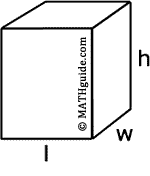MATHguide's Volume of Prisms Quizmaster
Review the Lesson | MATHguide homepage Updated June 18th, 2023

 Given the length (l) = 4 units, the width (w) = 5 units and the height (h) = 23 units: What is the area of the bottom? B = units2What is the volume? V = units3